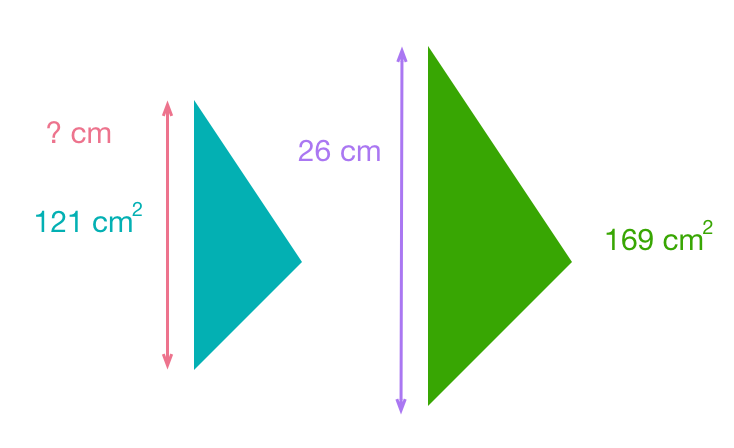# Everything Is Proportional

Geometry Level 1The area of two similar triangles is ${169\text{ cm}^2 }$ and ${121\text{ cm}^2}$ respectively. The length of the longest side of the larger triangle is $26\text{ cm}$. Find the length of the longest side of the smaller triangle.

×